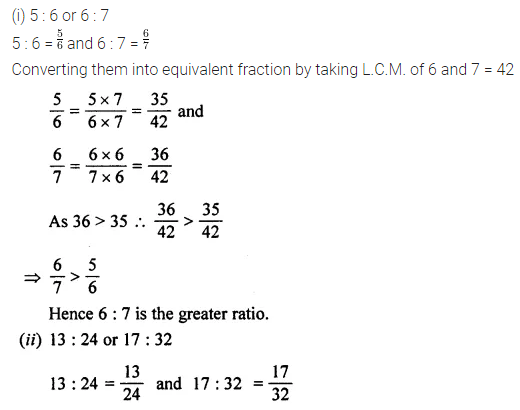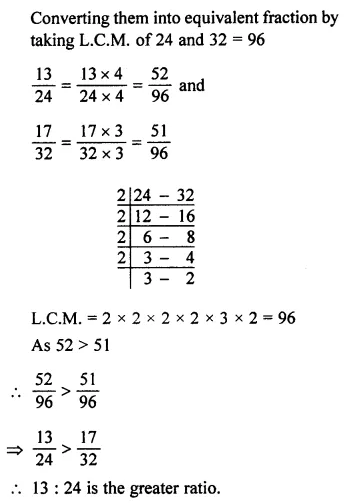# ML Aggarwal Class 7 Solutions for ICSE Maths Chapter 6 Ratio and Proportion Ex 6.1

## ML Aggarwal Class 7 Solutions for ICSE Maths Chapter 6 Ratio and Proportion Ex 6.1

Question 1.
Express the following ratios in simplest form:Solution: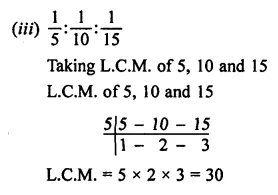Question 2.
Find the ratio of each of the following in simplest form:
(i) ₹ 5 to 50 paise
(ii) 3 km to 300 m
(iii) 9 m to 27 cm
(iv) 15 kg to 210 g
(v) 25 minutes to 1.5 hours
(vi) 30 days to 36 hours
Solution:Question 3.
If A : B = 3 : 4 and B : C = 8 : 9, then find A : C.
Solution: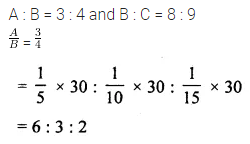Question 4.
If A : B = 5 : 8 and B : C = 18 : 25, then find A : B : C.
Solution:Question 5.
If 3A = 2B = 5C, then find A : B : C.
Solution:Question 6.
Out of daily income of ₹ 120, a labourer spends ₹ 90 on food and shelter and saves the rest. Find the ratio of his
(i) spending to income
(ii) saving to income
(iii) saving to spending.
Solution:Question 7.
5 grams of an alloy contains 3$$\frac { 3 }{ 4 }$$ grams copper and the rest is a nickel. Find the ratio by weight of nickel to copper.
Solution:Question 8.
A pole of height 3 meters is struck by a speeding car and breaks into two pieces such that the first piece is $$\frac { 1 }{ 2 }$$ of the second. Find the length of both pieces.
Solution: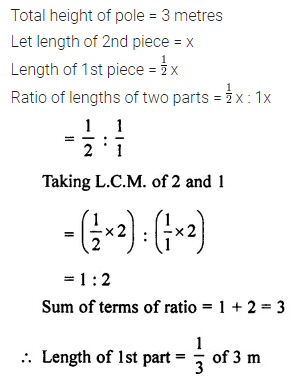Question 9.
Heights of Anshul and Dhruv are 1.04 m and 78 cm respectively. Divide 35 sweets between them in the ratio of their heights.
Solution: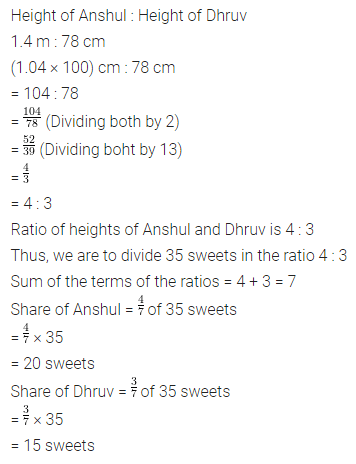Question 10.
₹ 180 are to be divided among three children in the ratio $$\frac { 1 }{ 3 } :\frac { 1 }{ 4 } :\frac { 1 }{ 6 }$$ Find the share of each child.
Solution: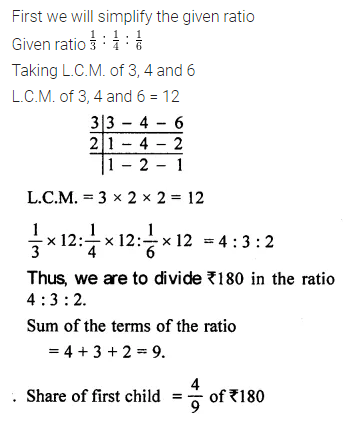Question 11.
A natural number has been divided into two parts in the ratio 7 : 11. If the difference between the two parts is 20, find the number and the two parts.
Solution: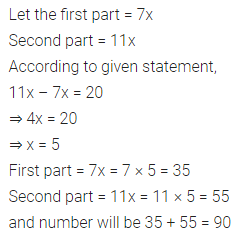Question 12.
A certain sum of money has been divided into two parts in the ratio 9 : 13. If the second part is ₹ 260, find the total amount.
Solution: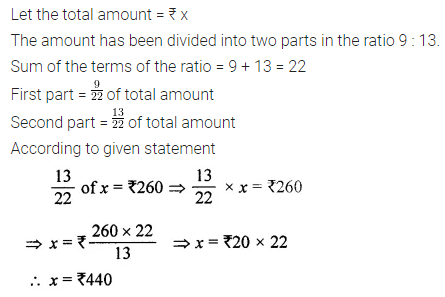Question 13.
The ratio of the present ages of Anjali and Ashu is 2 : 3. Five years hence, the ratio of their ages will be 3 : 4. Find their present ages.
Solution: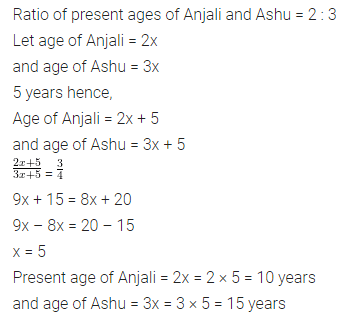Question 14.
The present ages of A and B are in the ratio 5 : 6. Three years ago, their ages were in the ratio 4 : 5. find their present ages.
Solution: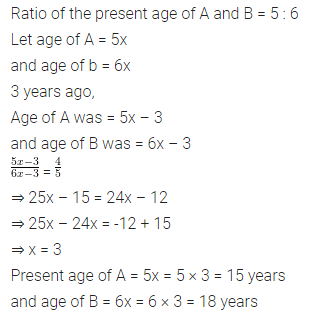Question 15.
Two numbers are in the ratio 5 : 6. When 2 is added to first and 3 is added to the second, they are in the ratio 4 : 5. Find the numbers.
Solution: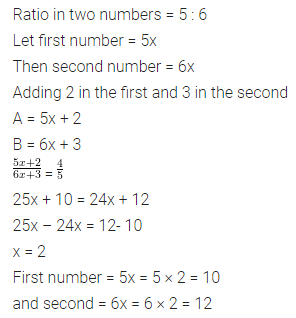Question 16.
The ratio of number of boys to the number of girls in a school of 1430 students is 7 : 6. If 26 new girls are admitted in the school, find how many new boys may be admitted so that the ratio of number of boys to the number of girls may change to 8 : 7.
Solution: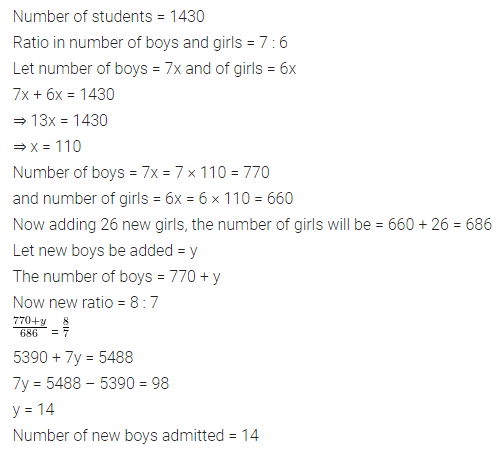Question 17.
Which ratio is greater?
(i) 5 : 6 or 6 : 7
(ii) 13 : 24 or 17 : 32
Solution: